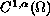Electron. J. Diff. Eqns., Vol. 2004(2004), No. 16, pp. 1-11.

### The eigenvalue problem for a singular quasilinear elliptic equation Benjin Xuan

Abstract:
We show that many results about the eigenvalues and eigenfunctions of a quasilinear elliptic equation in the non-singular case can be extended to the singular case. Among these results, we have the first eigenvalue is associated to aeigenfunction which is positive and unique (up to a multiplicative constant), that is, the first eigenvalue is simple. Moreover the first eigenvalue is isolated and is the unique positive eigenvalue associated to a non-negative eigenfunction. We also prove some variational properties of the second eigenvalue.

Submitted August 15, 2003. Published February 6, 2004.
Math Subject Classifications: 35J60.
Key Words: Singular quasilinear elliptic equation, eigenvalue problem, Caffarelli-Kohn-Nirenberg inequality.

Show me the PDF file (225K), TEX file, and other files for this article.Benjin Xuan Department of Mathematics University of Science and Technology of China Dept. de Matematicas, Universidad Nacional Bogota, Colombia e-mail: wenyuanxbj@yahoo.com